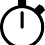### Temperature Basics: Mastering the Thermometer

One part of all basic math courses, including those designed for fifth graders, is learning how to master a thermometer. By fifth grade your child will know how to read the temperature on the thermometer, but he might not know how to convert Fahrenheit to Celsius and vice versa. For Celsius to Fahrenheit you'll need to take the given Celsius and multiply it by 1.8. Take the answer and add 32 to it. This is the temperature in Fahrenheit. For the reverse conversion, you'll need to take the given Fahrenheit and subtract 32 from it. The answer must then be divided by .556. The answer is your temperature in Celsius.

1.2.3.